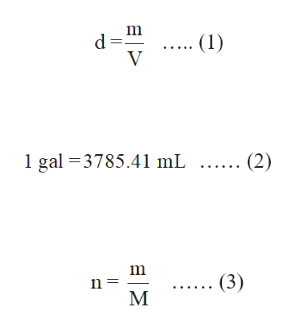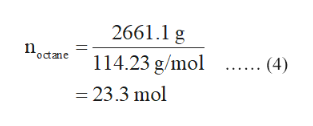How many kg of CO2 are produced when 1.00 galloon of octane (C8H18, density = 0.703 g/mL) is completely combusted in the presence of O2 to form CO2 and H2O?

Question

How many kg of CO2 are produced when 1.00 galloon of octane (C8H18, density = 0.703 g/mL) is completely combusted in the presence of O2 to form CO2 and H2O?

Step 1

Density of a substance is the ratio of its mass and volume. It is expressed in equation (1) in which d is the density, m is the mass and V is the volume.

The relation between gallon (gal) and millilitres (mL) is given in equation (2).

The number of moles is the ratio of given mass by molar mass. It is expressed in equation (3) in which n is the number of moles, m is the given mass and M is the molar mass.help_outlineImage Transcriptionclose= P V (1) 1 gal 3785.41 mL (2) . (3) М fullscreen
Step 2

The balanced reaction of complete combustion of octane is written below:

2C8H18(g)+25O2(g) →16CO2(g)+18H2O(g)

From equation (2), 1 gal of octane is equal to 3785.41 mL . The mass of octane is calculated by substituting 3785.41 mL for V and 0.703 g/mL for d in equation (1).

Step 3

The number of moles of octane is calculated is shown in equation (4) by usin...help_outlineImage Transcriptionclose2661.1 g octane 114.23 g/mol (4) =23.3 mol fullscreen

Want to see the full answer?

See Solution

Want to see this answer and more?

Our solutions are written by experts, many with advanced degrees, and available 24/7

See Solution
Tagged in

General Chemistry